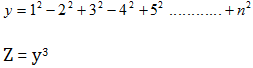# Create run shortcut for your Favorite File

When you need your favorite file to open what do you do? Usually you go to that directory and then open that file. After a certain period of time you get bored of this and ask yourself isn’t there any way to open my most favorite file easily? My answer will be yes.

Here today I will introduce a way through which you will be able to open your favorite file just writing its name in the run command. You just have to follow some easy steps before you are done.

# Some Java program part 6

1. Write a Java program that would input three numbers from the user and print sum, then the first number, then the 2nd number followed by 3rd number. If user enters 10, 20, 30. then output should be 60, 10, 20, 30.
```import java.util.Scanner;
public class Lab8q4{
public static void main ( String [] args ){
int [] number = new int ;
int c = 0, sum=0;
Scanner input = new Scanner ( System.in );
System.out.println(&quot;Enter 3 numbers&quot;);
while(c&lt;3){
number  = input.nextInt();
sum = sum + number ;
++c;
}
System.out.println(&quot;SUM : &quot;+sum);
c=0;
while(c&lt;3){
System.out.println(number);
++c;
}
}
}
```
1. Write a Java program that would input ten numbers from the user and print the ten numbers in reverse order. If user enters 20, 10, 30, 15. Then output should be 15, 30, 10, 20.
```import java.util.Scanner;
public class Lab8q5{
public static void main ( String [] args ){
int [] number = new int ;
int c = 0, sum=0;
Scanner input = new Scanner ( System.in );
System.out.println(&quot;Enter 3 numbers&quot;);
while(c&lt;3){
number  = input.nextInt();
sum = sum + number ;
++c;
}
c=2;
while(c&gt;=0){
System.out.println(number);
--c;
}
}
}
```

# Some Java programs part-5

21. Write a program that will calculate the value of Z  if the expression of y is as follows (n is the input):# Some Java programs part 4

16. Write a program that will read 10 numbers from the user, and then print the first number, the sum of the first 2 numbers, first 3 numbers, and so on up to the sum of 20 numbers.

```/*Time : 5:00pm
* Author: Anik das*/
import java.util.Scanner;
public class Faraz16{
public static void main ( String [] args ){
int c=1, sum=0, number;
Scanner input = new Scanner ( System.in );
while (c&lt;=10){
System.out.println(&quot;Enter Number #&quot;+c);
number = input.nextInt();
sum=sum+number;
System.out.println(&quot;Sum Of 1st &quot;+c+&quot; Number(s) is : &quot;+sum);
c++;
}
}
}
```